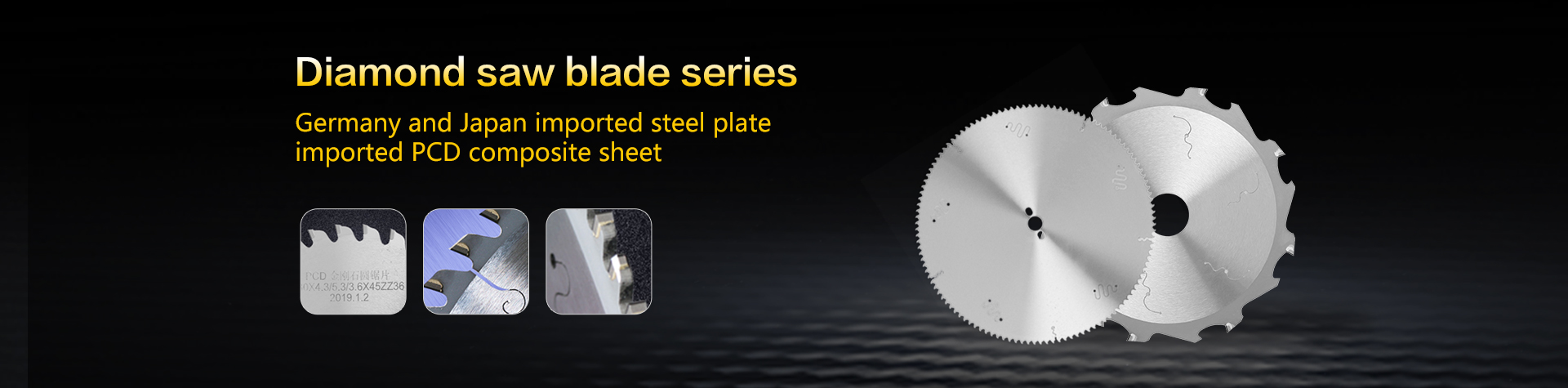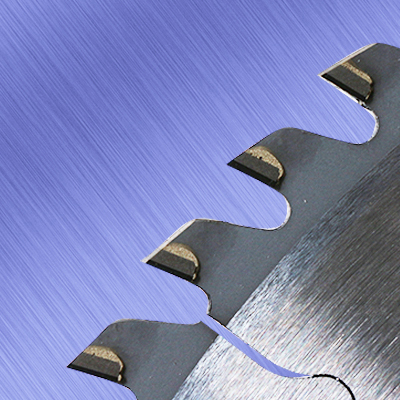### Related news

No search results found！

# How to calculate the operating speed of diamond saw blade 2020-06-18 11:34:43

The feed speed of the metal circular saw blade is what we often say the running speed, which can affect the output of the company's sawing, so we also need to know the feed speed of the metal circular saw blade clearly. There are two calculation methods below For reference.

1. Formula calculation method

A. The formula for the feed rate of metal circular saw blades is: feed rate = n * Z * feed amount (n represents the speed per minute, Z represents the number of teeth).

B. Example explanation:

hypothesis

Feed per tooth=0.08 mm/tooth

Number of teeth Z = 180 teeth

N =150rpm

Apply the formula to get: 150*180*0.08=2160(mm/min).

Second, use the counter to calculate the feed speed of the metal circular saw blade

A、Method introduction:

1. Hold down the stopwatch when you hear the sound of the saw blade cutting into the workpiece.

2. Stop the stopwatch when the sawing sound stops.

Recommend to test a few times, and then take an average, this will be more accurate.

B. Formula application and case:

1. Formula application: feed rate = (material diameter x60) / sawing time.

This formula is completely different from the above formula. This is based on the actual sawing time, which can be said to be closer to our needs.Related tags：